nextnano.com  GUI: nextnanomat  Tool: nextnano++  Tool: nextnano³  Tool: nextnano.NEGF  Download | Search | Copyright | News | Publications  * password protected nextnano³ software2D T-shaped QWR

# nextnano3 - Tutorial

## Electron and hole wave functions in a T-shaped quantum wire grown by CEO (cleaved edge overgrowth)

Author: Stefan Birner

If you want to obtain the input files that are used within this tutorial, please contact stefan.birner@nextnano.de.
` -> 2DTshapedQuantumWireCEO.in`

Acknowledgement: The author - Stefan Birner - would like to thank Robert Schuster from the University of Regensburg for providing the experimental data and some of the figures.

## T-shaped quantum wire

Similar to the 1D confinement in a quantum well, it is possible to confine electrons or holes in two dimensions, i.e. in a quantum wire.
The quantum wire is formed at the T-shaped intersection of two 10 nm GaAs type-I quantum wells and is confined by Al0.35Ga0.65As barriers. The electrons and holes are free to move along the z direction only, thus the wire is oriented along the [0-11] direction. Such a heterostructure can be manufactured by growing the layers along two different growth directions with the CEO (cleaved egde overgrowth) technique. Due to the nearly identical lattice constants of GaAs and AlAs it is possible to assume this heterostructure as being unstrained.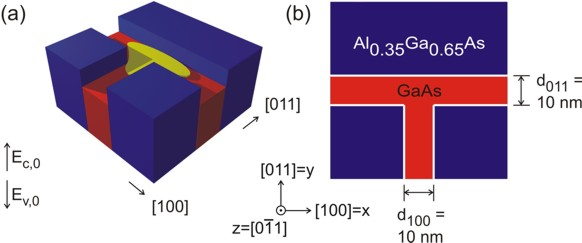The figure on the left shows schematically the conduction band profile of the T-shaped wire that was grown by the CEO technique. If one inverts the energy arrow then the left picture corresponds to the valence band edge. The wave function is indicated at the T-shaped intersection in yellow. Here, the wave function can extend into a larger volume (as compared to the quantum well) and thus reduce its energy. So quantum mechanics tells us that the ground state can be found at this intersection and electrons are only allowed to move one-dimensionally along the z direction. The figure on the right shows a 60 nm x 60 nm extract of the schematic layout including the dimensions, the material composition and the orientation of the wire with respect to the crystal coordinate system.

It is sufficient to describe this heterostructure within a 2D simulation as it is translationally invariant along the z direction.
The simulation coordinate system is oriented in the following way:
```  \$domain-coordinates   domain-type        = 1  1  0  ! ```(x,y) plane```   hkl-x-direction-zb = 1  0  0  ! ```along the`   `direction in the crystal coordinate system```   hkl-y-direction-zb = 0  1  1  ! ```along the`   `direction in the crystal coordinate system``` ! hkl-z-direction-zb = 0 -1  1  ! ``` along the` [0-11] `direction in the crystal coordinate system``` ```The` hkl-z-direction `does not have to be specified. It is calculated internally in the code.

The simulations are performed at 4 Kelvin:
``` \$global-parameters   lattice-temperature = 4.0d0   ! [K]```

### Electron, heavy hole and light hole wave functions

The electron and hole wave functions can be calculated within the effective mass theory (envelope function approximation) by using position dependent effective masses. In our example, the effective masses are constant within each material but have discontinuities at the material interfaces. For the electrons, the effective mass was assumed to be isotropic (conduction band minimum at the Gamma point) whereas for the heavy and light holes we used anisotropic effective mass tensors that were derived from the 6-band k.p parameters (or Luttinger parameters).

The Luttinger parameters for GaAs are given by:
`- gamma1 = 6.98                               ` AlAs:``` 3.76 - gamma2 = 2.06                               ``` AlAs:``` 0.82 - gamma3 = 2.93                               ``` AlAs:` 1.42`

The effective masses of GaAs in units of [m0] can thus be calculated from these Luttinger parameters for different directions:
`- `electron (Gamma point):```                                                    0.067      ``` AlAs:` ```` 0.15```
`- `heavy hole along`  `direction:```  1 / ( gamma1 -   2  gamma2 )             = 0.350      ``` AlAs:``` 0.472```
`- `heavy hole along`  `direction:```  1 / ( gamma1 - 1/2 (gamma2 + 3 gamma3) ) = 0.643      ``` AlAs:` ```` 0.820```
`- `light   hole along`  `direction:```  1 / ( gamma1 +   2  gamma2 )             = 0.090      ``` AlAs:` ```` 0.185```
`- `light   hole along`  `direction:```  1 / ( gamma1 + 1/2 (gamma2 + 3 gamma3) ) = 0.081      ``` AlAs:` ```` 0.159 - ```heavy hole isotropic:                         ```1 / ( gamma1 - 0.8 gamma2 - 1.2 gamma3 ) = 0.551      ``` AlAs:` ```` 0. - ```light   hole isotropic:                         ```1 / ( gamma1 + 0.8 gamma2 + 1.2 gamma3 ) = 0.082      ``` AlAs:` ```` 0.```

Usually the database entries for the effective masses assume spherical symmetry for the holes and are specified with respect to the crystal coordinate system.
Their default values (isotropic) and the values which were derived from the Luttinger parameters are given in this table:

 heavy hole (GaAs) light hole (GaAs) heavy hole (AlAs) light hole (AlAs) along`  `direction `0.350` `0.090` ``` 0.472``` ``` 0.185``` along`  `direction `0.643` `0.081` ``` 0.820``` ``` 0.159``` isotropic `0.551` `0.082` `0.714` `0.163` nextnano³ database (default) `0.500` `0.068` `0.5` `0.26`

In this tutorial, however, we calculated the effective masses for different directions and therefore we do not have spherical symmetry anymore.
Thus we have to rotate the new eigenvalues of the effective mass tensors that are given in the x=, y=, z=[0-11] simulation coordinate system into the crystal coordinate system where xcr=, ycr=, zcr=.
First we have to overwrite the default entries in the database so that they contain the eigenvalues of the effective mass tensors in the simulation system.```  conduction-band-masses   = 0.067d0  0.067d0  0.067d0 ! ```electron effective mass (Gamma point) (default)```                             ...`````` `````` valence-band-masses      = 0.350d0  0.643d0  0.643d0 ! ```eigenvalues of the heavy hole effective mass tensor    [0-11]```                             0.090d0  0.081d0  0.081d0 ! ``` eigenvalues of the light   hole effective mass tensor    [0-11]```                             ...                       ! ```To project these eigenvalues onto the crystal coordinate system we need to know the principal axes system which these eigenvalues refer to.```  principal-axes-vb-masses = 1d0      0d0      0d0     ! ```heavy hole  ```                             0d0      1d0      1d0     !         ``` ```                             0d0     -1d0      1d0     !         ``` [0-11]```                             1d0      0d0      0d0     ! ```light hole    ```                             0d0      1d0      1d0     !         ``` ```                             0d0     -1d0      1d0     !         ``` [0-11]```                             ... ```(The normalization of these vectors will be done internally by the program.)

Similar, the valence band masses of AlAs are chosen to be:
```  conduction-band-masses   = 0.15d0   0.15d0   0.15d0  ! ```electron effective mass (Gamma point) (default)```  valence-band-masses      = 0.472d0  0.820d0  0.820d0 ! ```eigenvalues of the heavy hole effective mass tensor    [0-11]```                             0.185d0  0.159d0  0.159d0 ! ``` eigenvalues of the light   hole effective mass tensor    [0-11]

Further material parameters of relevance:

Conduction band offset Al0.35Ga0.65As / GaAs:  0.2847 eV
Valence     band offset Al0.35Ga0.65As / GaAs: -0.1926 eV
Egap Al0.35Ga0.65As: 2.2883 eV
Egap GaAs:              1.5193 eV

As we do not have doping and no piezoelectric fields (the structure is assumed to be unstrained) and as the temperature is assumed to be 4 K, we do not have to deal with charge redistributions. Thus we can refrain from solving Poisson's equation and we also do not have to take care about self-consistency.

Both, the heavy hole and the light hole band edge energies are degenerate but the effective mass tensors differ. Thus we have to solve three Schrödinger equations, namely for the
- conduction band
- heavy hole band
- light   hole band
The lowest hole state is the heavy hole state and the second hole state is the light hole state. No further hole states are confined. Also, in the conduction band only the ground state is confined.

The following figures show the charge densities (Psi²; Psi = wave function) of the ground states of the confined electron, heavy and light hole eigenstates of the quantum wire.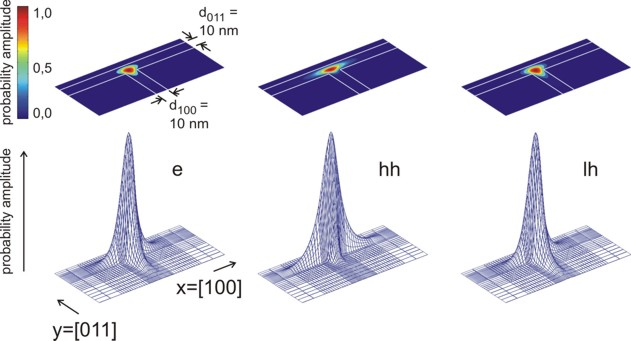The probabiliy amplitudes of the electron (e), the heavy hole (hh) and the light hole (lh) envelope functions at an unstrained T-shaped intersection of two 10 nm wide GaAs quantum wells embedded by Al0.35Ga0.65As barriers. For the lower three pictures, the wave functions are normalized so that the maximum of each equals one.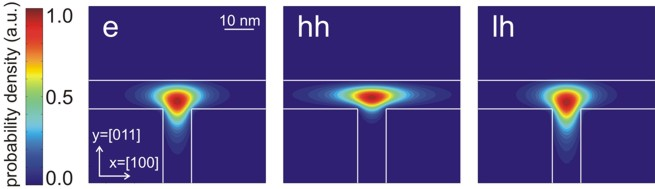Contour diagram of the probabiliy amplitudes of the electron (e), heavy hole (hh) and light hole (lh) eigenfunctions (same figures as the ones above but this time viewed from the top). One can clearly see that each ground state wave function is localized at the T-shaped intersection and shows the T-shaped symmetry. Due to the anisotropy of the heavy hole effective mass, the heavy hole wave function prefers to extend along the   direction and hardly penetrates into the quantum well that is aligned along the  direction. The heavy hole mass along the  direction is only half as heavy as along the  direction. The light hole anisotropy is only minor and thus its symmetry resembles the one of the isotropic electron. Again, the normalization is chosen so that the maximum of the wave function equals one.

The quantum cluster (`\$quantum-regions`) used in this calculation has the size 108 nm x 108 nm. (The whole simulation area is 110 nm x 110 nm.) The figures show an extract of 60 nm x 60 nm.

The calculated eigenvalues are:

Single-band Schrödinger equation (`effective-mass`)

 ```schroedinger-masses-anisotropic = ``` `box` `yes` `no` electron     ground state energy (eV) `3.00584185` `3.00584196` `3.00582875` heavy hole ground state energy (eV) `1.45511017` `1.45511001` `1.45511013` light   hole ground state energy (eV) `1.43906098` `1.43906078` `1.43906813`

6-band k.p Schrödinger equation (`6x6kp`)

 ```schroedinger-kp-discretization = ``` ``` box-integration``` ``` box-integration``` ``` finite-differences``` ```kp-vv-term-symmetrization = ``` `no` `yes` `yes` 1st   hole energy (eV) (6-band k.p) `1.4549 `(2fold degenerate) `- `(2fold degenerate) `- `(2fold degenerate) 2nd  hole energy (eV) (6-band k.p) `- `(2fold degenerate) `- `(2fold degenerate) `- `(2fold degenerate) 3rd   hole energy (eV) (6-band k.p) `- `(2fold degenerate) `- `(2fold degenerate) `- `(2fold degenerate) 4th   hole energy (eV) (6-band k.p) `- `(2fold degenerate) `- `(2fold degenerate) `- `(2fold degenerate) 5th   hole energy (eV) (6-band k.p) `- `(2fold degenerate) `- `(2fold degenerate) `- `(2fold degenerate)

Note:

• The grid lines have an equally spaced separation of 1 nm.
Choosing a denser gridding of 0.5 nm separation between 25 and 85 nm leads to the following eigenvalues for` box`:
`3.00529`, `1.45525`, `1.43942`.
• Conduction band edge energy (GaAs) = 2.979 eV, hole band edge energy (GaAs) = 1.459667 eV.
• Neumann boundary conditions were used. In case of confinement, the wave function is zero at the quantum cluster boundaries.

(Here,``` schroedinger-masses-anisotropic = no ```leads to the same results as the mixed derivatives d²/(dxdy) are zero because our effective mass tensors are either isotropic (electrons) or oriented with their principal axes parallel to the simulation system (holes).)

For these calculations, we neglected excitonic effects.
`  => `electron - heavy hole transition energy = 1.551 eV
`  => `electron - light   hole transition energy = 1.567 eV

AVS/Express screenshots where the scale of the wave function (psi²) is given in 1/nm². Integration of psi² over the simulation area of 110 * 110 nm² sums up to one.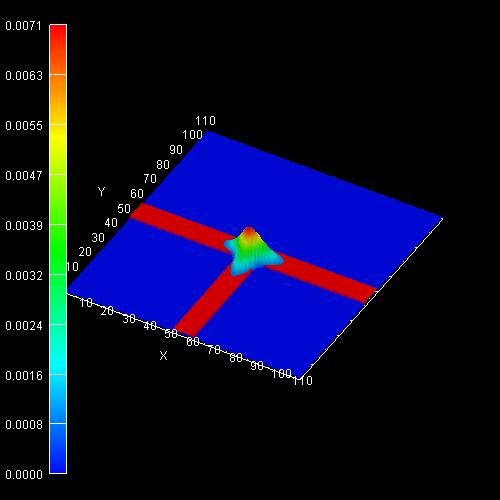In addition to these ground states for kz=0, excited states are possible as well. Similar to the subbands of a 1D quantum well that show a E(kx,ky) dispersion one can assign a subband with the energy dispersion E(kz) to each quantum wire eigenvalue which describes the free motion along the quantum wire axis (z axis). A more advanced treatment would be to use k.p theory to calculate the eigenvalues for different kz in order to obtain the (nonparabolic) energy dispersion E(kz).

Understanding the meaning of the 6-band k.p parameters

For the same structure as above we perform the calculations again but this time using 6-band k.p instead of single-band.

The left figure shows the wave function (psi²) for the hole ground state where we used for GaAs the same Luttinger parameters as above:
` gamma1 = 6.98`,` gamma2 = 2.06`,``` gamma3 = 2.93 ```(This corresponds to:` L = -16.220`,``` M = -3.860```,` N = -17.580`)

The right figure shows the wave function (psi²) for the hole ground state where we used for GaAs the Luttinger parameters
` gamma1 = 6.98`,``` gamma2 = 2.06 = gamma3 ```(This corresponds to:` L = -16.220`,``` M = -3.860```,` N = -12.36`)
Choosing` gamma2 = gamma3 `corresponds to an isotropic effective mass.

These results are in very good qualitative agreement with the heavy hole and light hole wave functions calculated within the single-band approach. The impact of an isotropic (for electrons and light holes) or anisotropic (for heavy hole) effective mass tensor should now be clear.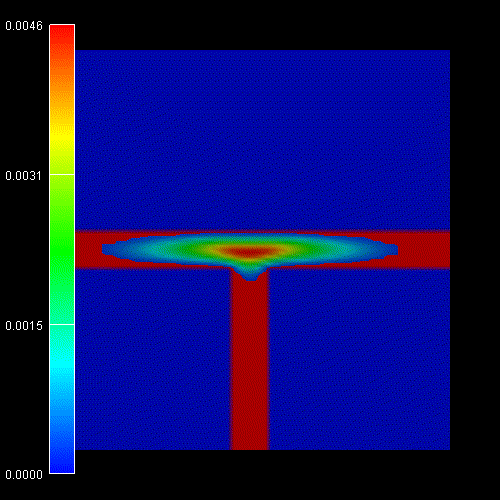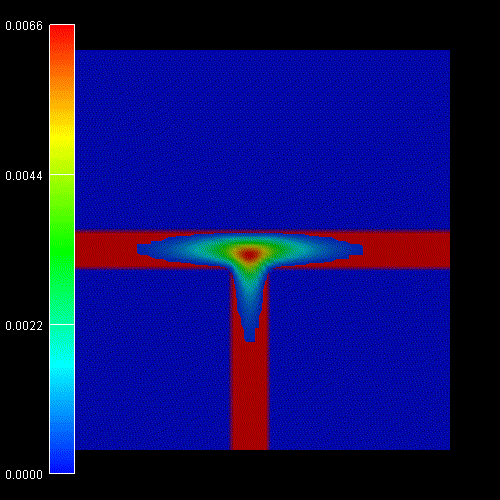Within the 6-band k.p calculations we get only one confined hole state, in contrast to the single-band effective-mass approach where we obtained one confined state for the heavy hole and another confined state for the light hole.

Note: Here we used Dirichlet boundary conditions.

### Interband transitions

(Note: This part has to be updated: Now we output the square of this matrix element.)

We evaluate the spatial overlap integral between the electron and hole envelope wave functions of the ground states. These numbers are proportional to the transition probability. Additionally, the polarization of light has to be taken into account to get the correct probability.

``` \$output-1-band-schroedinger   ...   interband-matrix-elements = yes```

The results are contained in these files:
```  Schroedinger_1band/interband2D_vb001_cb001_qc001_hlsg001_deg001_neu.dat ! ```heavy hole` <-> `electron```   Schroedinger_1band/interband2D_vb002_cb001_qc001_hlsg002_deg001_neu.dat ! ```light   hole` <-> `electron

`vb001`: valence band` 1`, i.e. heavy hole``` vb002```: valence band` 1`, i.e. heavy hole
`cb001`: conduction band` 1`, i.e. electron for Gamma point

``` heavy hole <-> Gamma conduction band                         matrix element     transition energy e1-hh1  ------------------------------------------------------------------  <psi_vb001|psi_cb001>   0.9048                           1.5507 eV```

``` light hole <-> Gamma conduction band                         matrix element     transition energy e1-lh1  ------------------------------------------------------------------  <psi_vb001|psi_cb001>   0.9946                           1.5668 eV```

`vb001`: valence band hole state` 1`, i.e. (heavy or light) hole ground state``` cb001```: conduction band state `1`, i.e. electron ground state

By looking at the wave functions (psi²), one would expect a larger overlap between the electron and the light hole rather than electron / heavy hole.
As the calculatations show, this is exactly the case: The electron / heavy hole overlap is only` 0.9 `whereas the electron / light hole overlap is much larger:``` 0.99```.

Note: A comparison of these results with different eigenvalue solvers and discretization routines:

``` heavy hole <-> Gamma conduction band                         matrix element     transition energy e1-hh1  ------------------------------------------------------------------  <psi_vb001|psi_cb001>  -0.904760286602367 (box) 1.550732 eV                          0.904842702923420 (yes) 1.550732 eV                          0.904765324859528 (no)  1.550719 eV                                            (chearn,box)                          0.556467704746565 (chearn,yes) 1.550732 eV -> imaginary part of psi is NOT zero                         -0.904765324861447 (chearn,no)  1.550719 eV                         -0.574566655967155 (arpack,box) 1.550732 eV -0.574531849467085 (bug fix diag_sgV)                          0.556467704746344 (arpack,yes) 1.550732 eV -> imaginary part of psi is NOT zero -> complex arpack                          0.904765324861290 (arpack,no)  1.550719 eV                          0.904765324859528 (no,magnetic) 1.550719 eV -> imaginary part of psi is zero                          0.556442520299478 (chearn,no,magnetic) 1.550719 eV -> imaginary part of psi is NOT zero                          0.556442520299654 (arpack,no,magnetic) 1.550719 eV -> imaginary part of psi is NOT zero -> complex arpack  ```

``` light hole <-> Gamma conduction band                         matrix element     transition energy e1-lh1  ------------------------------------------------------------------  <psi_vb001|psi_cb001>   0.994609977897094 (box) 1.566781 eV                         -0.994618620870019 (yes) 1.566781 eV                          0.994618072678695 (no)  1.566761 eV                                            (chearn,box)                         -0.579198621913980 (chearn,yes) 1.566781 eV -> imaginary part of psi is NOT zero                          0.994618072678062 (chearn,no)  1.566761 eV                          0.181672284480707 (arpack,box) 1.566781 eV 0.181668617064867 (bug fix diag_sgV)                          0.665134365762336 (arpack,yes) 1.566781 eV -> imaginary part of psi is NOT zero -> complex arpack                         -0.994618072678148 (arpack,no)  1.566761 eV                          0.994618072678695 (no,magnetic) 1.56676  eV -> imaginary part of psi is zero                         -0.579560683962475 (chearn,no,magnetic) 1.566761 eV -> imaginary part of psi is NOT zero                         -0.579560683962440 (arpack,no,magnetic) 1.56676  eV -> imaginary part of psi is NOT zero -> complex arpack ```See` \$output-1-band-schroedinger ` for an explanation of this.

• Please help us to improve our tutorial. Send comments to``` support [at] nextnano.com```.